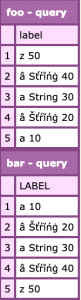Sorting text is a common task and can be done when getting data, such as from a database, or once we have the data using in-built functions such as arraySort.

Here’s a quick example:

```foo = [
"z 50",
"a String 40",
"a String 30",
"a String 20",
"a 10"
];

bar = foo.sort("textnocase");```

The value of `bar` is as we’d expect:

```[
"a 10",
"a String 20",
"a String 30",
"a String 40",
"z 50"
]
```

Things get a bit more complicated when you have strings containing characters with diacritical marks. For example:

```foo = [
"z 50",
"â Sťŕĭńġ 40",
"a String 30",
"â Šťŕĭńġ 20",
"a 10"
];
```

When we sort this array as we did before with `arraySort` we end up with this:

```[
"a 10",
"a String 30",
"z 50",
"â Sťŕĭńġ 40",
"â Šťŕĭńġ 20"
]
```

This is probably not what we want. Let’s try using our own sorting function.

```function sortAZ(first, second) {
return compareNoCase(first, second);
}

foo = [
"z 50",
"â Sťŕĭńġ 40",
"a String 30",
"â Šťŕĭńġ 20",
"a 10"
];

bar = foo.sort(sortAZ);
```

Unfortunately we get the same result.

Fortunately this isn’t a new problem and Java includes some methods to help us – enter the `java.text.Normalizer` which was added in Java 1.6.

`java.text.Normalizer` allows us to normalise (or normalize if you prefer!) text so that it can be sorted or searched by converting the string to something that can be compared. It supports four Unicode text normalization forms; NFC, NFD, NFKC and NFKD. You can read more about them in the docs https://docs.oracle.com/javase/tutorial/i18n/text/normalizerapi.html

So how we can use it in our CFML code? The following examples were written for ColdFusion 2018 Update 2 but essentially the code is the same on older versions and other engines.

```function TextNormalizer() {
var Normalizer = new java("java.text.Normalizer");
// ENUM
var NormalizerForm = new java("java.text.Normalizer\$Form");

return function(input) {
return Normalizer.normalize(input, NormalizerForm.NFKD);
}
}

normalize = TextNormalizer();

function sortAZ(first, second) {
return compareNoCase(
normalize(first),
normalize(second)
);
}

foo = [
"z 50",
"â Sťŕĭńġ 40",
"a String 30",
"â Šťŕĭńġ 20",
"a 10"
];

bar = foo.sort(sortAZ);
```

Running this produces the following:

```[
"a 10",
"a String 30",
"â Sťŕĭńġ 40",
"â Šťŕĭńġ 20",
"z 50"
]
```

It’s much better – we have `a` at the top and `z` at the bottom. We’re still not quite there yet. After some Googling I came across this page https://www.regular-expressions.info/unicode.html which had this snippet:

`\p{M}` or `\p{Mark}`: a character intended to be combined with another character (e.g. accents, umlauts, enclosing boxes, etc.).

Adding that into the mix we get this:

```function TextNormalizer() {
var Normalizer = new java("java.text.Normalizer");
// ENUM
var NormalizerForm = new java("java.text.Normalizer\$Form");

return function(input) {
var result = Normalizer.normalize(input, NormalizerForm.NFKD);
return result.replaceAll("\p{M}+", "");
}
}

normalize = TextNormalizer();

function sortAZ(first, second) {
return compareNoCase(
normalize(first),
normalize(second)
);
}

foo = [
"z 50",
"â Sťŕĭńġ 40",
"a String 30",
"â Šťŕĭńġ 20",
"a 10"
];

bar = foo.sort(sortAZ);
```

When we run this the result is:

```[
"a 10",
"â Šťŕĭńġ 20",
"a String 30",
"â Sťŕĭńġ 40",
"z 50"
]
```

Which is the result I was aiming for.

—-

So that’s how you sort an array, but sometimes you want to sort a query recordset in memory. Using `QuerySort` we can use the same technique to sort the records.

```function TextNormalizer() {
var Normalizer = new java("java.text.Normalizer");
// ENUM
var NormalizerForm = new java("java.text.Normalizer\$Form");

return function(input) {
var result = Normalizer.normalize(input, NormalizerForm.NFKD);
return result.replaceAll("\p{M}+", "");
}
}

normalize = TextNormalizer();

function sortAZ(first, second) {
return compareNoCase(
normalize(first.label),
normalize(second.label)
);
}

foo = QueryNew("label",
"varchar",
[
["z 50"],
["â Sťŕĭńġ 40"],
["a String 30"],
["â Šťŕĭńġ 20"],
["a 10"]
]
);

bar = foo.duplicate().sort(sortAZ);

WriteDump(var=foo, label="foo");
WriteDump(var=bar, label="bar");
```

Note that `QuerySort` unlike `ArraySort` mutates the original variable, so I had to duplicate it first. Personally I think QuerySort should return a new query object, but hey-ho.

Here’s the query objects before and after.—-

One thing, I did try that didn’t work, was to use CFML’s `result.reReplace("\p{M}+", "", "all")` instead of the java string method `result.replaceAll("\p{M}+", "");`. The output from that was:

```[
"a 10",
"a String 30",
"â Sťŕĭńġ 40",
"â Šťŕĭńġ 20",
"z 50"
]
```

I’m not sure why that doesn’t work, but as I noted above java’s `replaceAll` worked, so I’m just going to use that.

—-

It’s also worth checking out this blog post by James Moberg where he recommends using `junidecode` to do this which isn’t part of Java.

1 Comment
2019-02-27 01:46:49

Great post, very useful… Thanks!